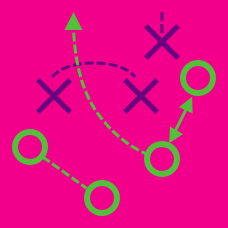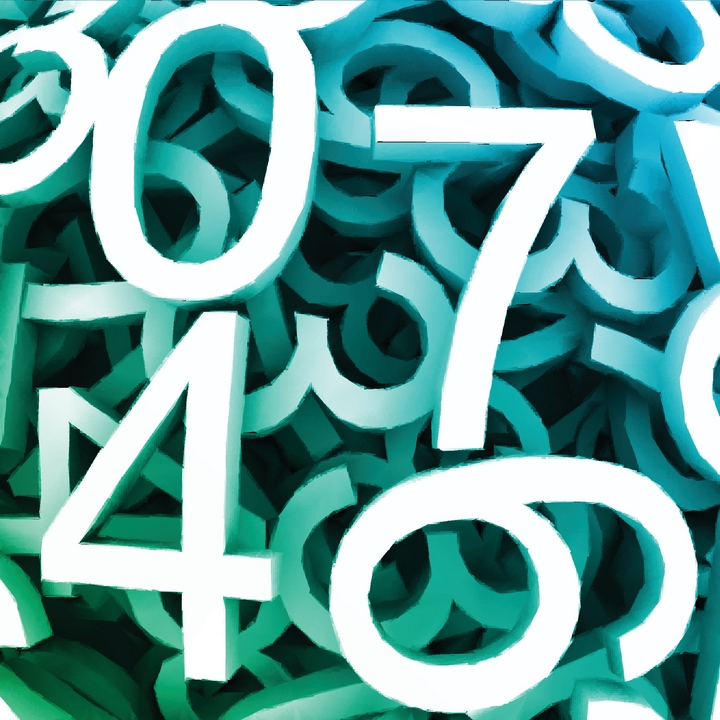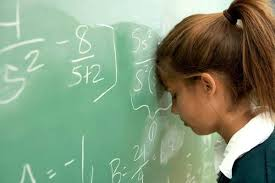Probability

# Problem Solving Tactics: Level 3 ChallengesConsider the set

$S= \left \{ 1, \frac {1}{2}, \frac {1}{3}, \frac {1}{4},\cdots, \frac {1}{100} \right \}.$

Choose any two numbers $x$ and $y,$ and replace them with $x+y+ xy.$

For example, if we choose the numbers $\frac{1}{2}$ and $\frac {1}{8}$, we will replace them by $\frac {11}{16}$.

If we keep repeating this process until only $1$ number remains, what is the final number?

There are 100 runners, each given a distinct bib labeled 1 to 100. What is the most number of runners that we could arrange in a circle, such that the product of the numbers on the bibs of any 2 neighboring runners, is less than 1000?

Some unit squares of a $2013 \times 2013$ grid are marked so that any $19 \times 19$ subgrid has at least $21$ marked unit squares. What is the minimal possible number of marked unit squares?

Find the largest positive integer $n<100,$ such that there exists an arithmetic progression of positive integers $a_1,a_2,...,a_n$ with the following properties.

1) All numbers $a_2,a_3,...,a_{n-1}$ are powers of positive integers, that is numbers of the form $j^k,$ where $j\geq 1$ and $k\geq 2$ are integers.

2) The numbers $a_1$ and $a_{n}$ are not powers of positive integers.Sergei chooses two different natural numbers $a$ and $b$. He writes four numbers in a notebook: $a$, $a+2$, $b$ and $b+2$.

He then writes all six pairwise products of the numbers of notebook on the blackboard.

What is the maximum number of perfect squares on the blackboard?

Assumption: Natural numbers don't include zero.

×